;nbsp

Hi parents and students! This is an introduction to what you’ll be needing to know by the end of the first PreCalc semester. My courses cover all of the common core requirements (the syllabus for semester 1 is here, and semester 2 is here!) and prepare my students to think critically. Not only do I want your student to know the material in my class, I want them to succeed in all future classes and opportunities. Please try out this sample test to see what your child will be learning this semester!

Name*

This is a required question

Email*

Must be a valid email address

1. Identify the 30-60-90 triangle with the correctly labeled sides of “standard length”
This is a required question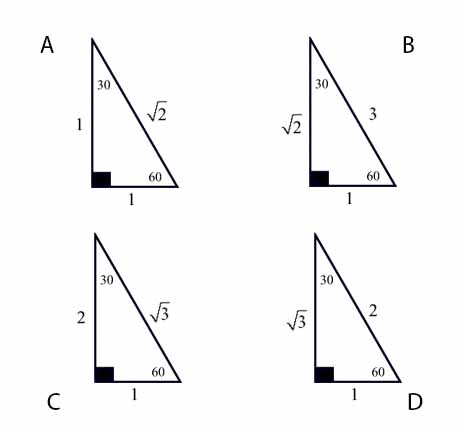2. Find tan(B/2) when sinB = -2/5, 3π/2 ≤ B ≤ 2π
This is a required question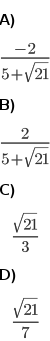3. Find cos(A/2) when cosA = -4/5, π/2 ≤ A ≤ π.
This is a required question

4. Determine the domain, x-intercept, and y-intercept for y = (x + 2)/(x² – 16)
This is a required question

5. Solve 2cosx – 5 + 2secx = 0.
Think of this as a quadratic! ax²+bx+c
This is a required question

6. Identify the 45-45-90 triangle with the correctly labeled sides of “standard length”
This is a required question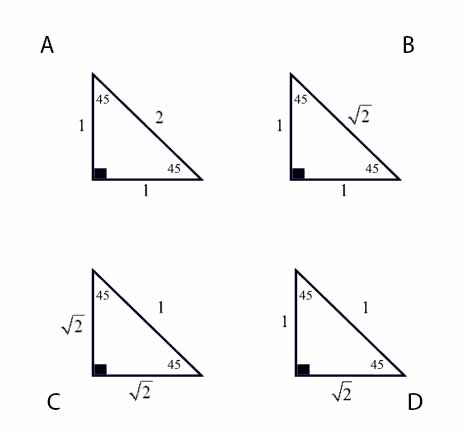7. Use the roots and end behavior to identify the graph of [f(x) = -x⁴ + 16x²]
This is a required question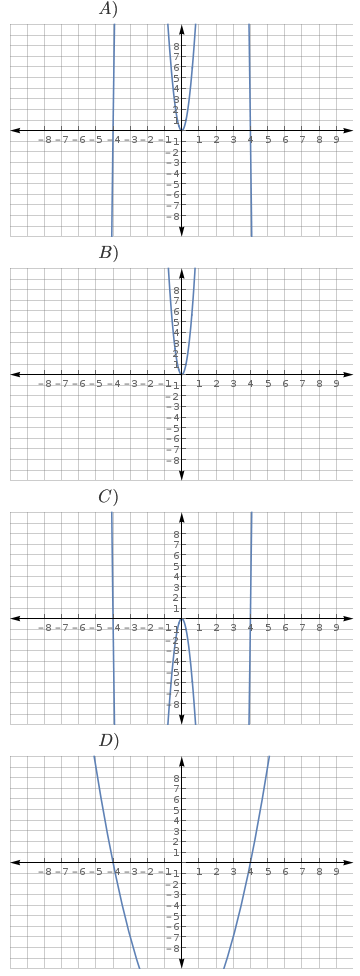8. Convert 3π/11 radians to degrees.
This is a required question

9. Find the horizontal and vertical components of a force vector having an angle of depression of 13° and a magnitude of 202 lb.
This is a required question

10. Find (f ○ g)(x) where [f(x) = 3x² + 1] & [g(x) = 1/(x+5)]
This is a required question

11. If cosθ = -5/13 and θ is in the 3rd quadrant, find sinθ and tanθ.
This is a required question

12. Two ropes are horizontally pulling on a tree The first has a magnitude of 320 newtons and the second, 560 newtons. The angle between the forces is 36°. What is the magnitude of the resultant and what angle does it make with the 560 newton force?
Parallelogram Method for adding vectors!
This is a required question

13. Solve triangle ABC where a = 5, c = 12, B = 28°.
This is a required question14. Based on the graph above, what is the function at x = -2?
This is a required question15. Use a calculator to find csc(7π / 9) radians.
This is a required question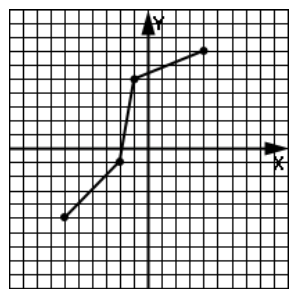16. Identify the reflection of this function across the y-axis:
This is a required question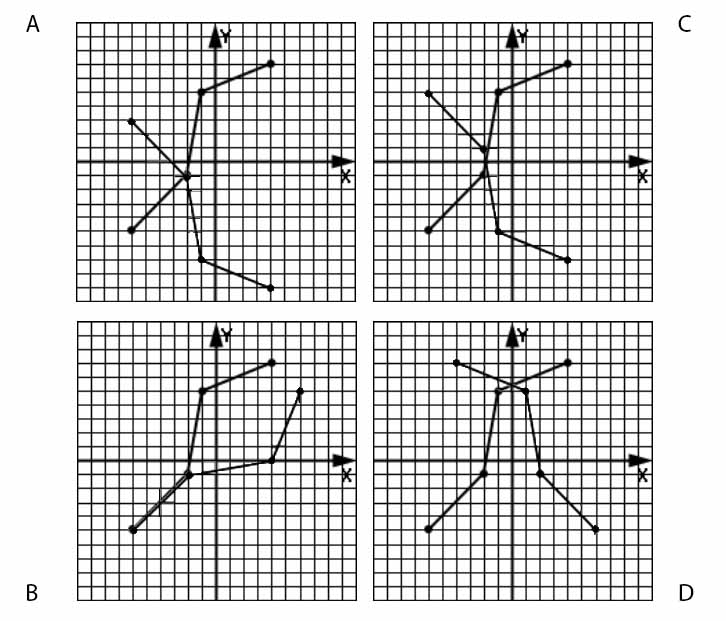17. Identify [f(x) = (25-x²)^(1/2) – 1]
This is a required question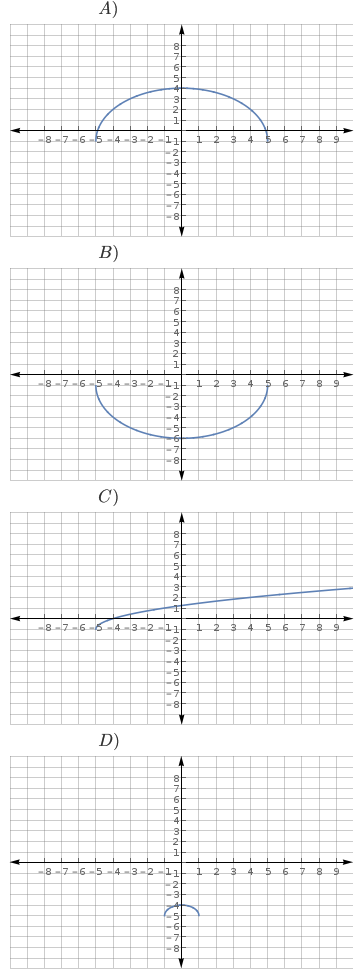18. Find the area of triangle ABC where A = 16°, B = 57° and a = 156.9.
Area = 1/2absinC, use Law of Sines to find your sides!
This is a required question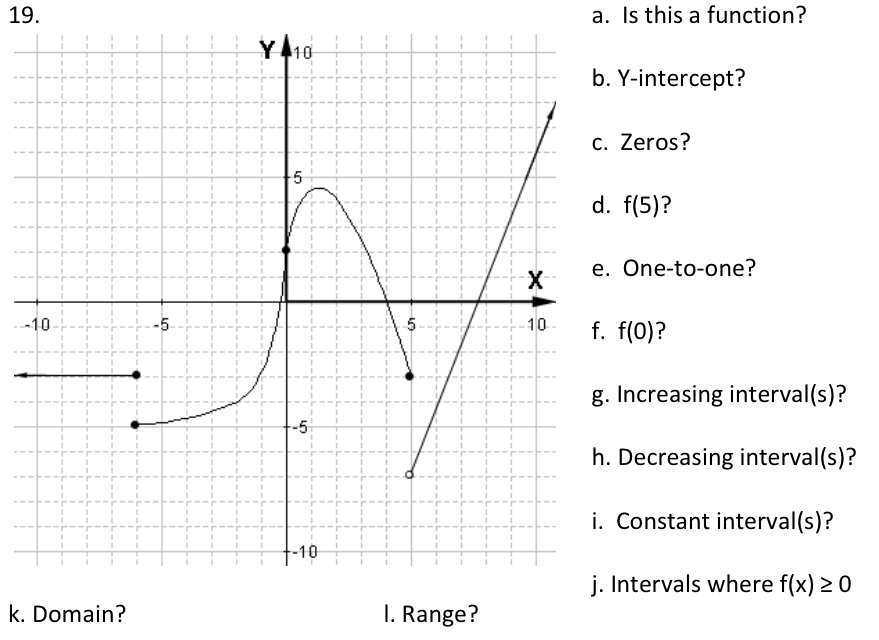19a. Is this a function? (Vertical Line Test)
This is a required question

19b. What are the Y-intercept(s)?
This is a required question

19c. What are the zeros?
This is a required question

19d. f(5) =
This is a required question

19e. Is the function one-to-one?
This is a required question

19f. f(0) =
This is a required question

19g. Increasing intervals
This is a required question

19h. Decreasing Intervals
This is a required question

19i. Constant Intervals
This is a required question

19k. Intervals where f(x) ≥ 0
This is a required question

20. Find f(-3) where f(x) = (6x – 2)/x²
This is a required question

21. Find sin(A + B) where sinA = -2/5, π ≤ A ≤ 3π/2; cosB = 1/5, 3π/2 ≤ B ≤ 2π.
This is a required question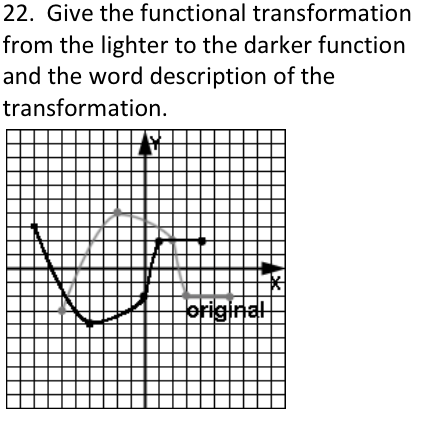22. What transformation occurs in the graph?
Start with the light gray graph, transform to the black graph.
This is a required question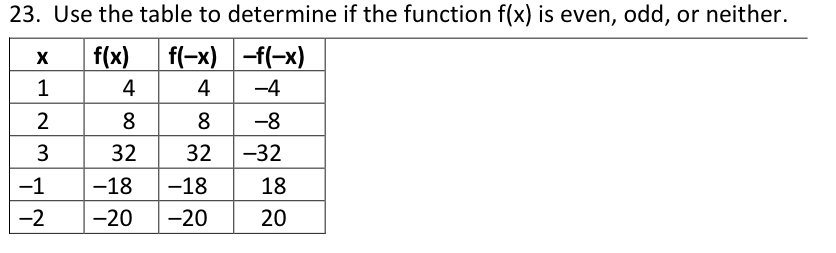23. Use the table to determine if the function f(x) is even, odd, or neither.
This is a required question

24. Convert 86.4781° to degrees, minutes, and seconds
This is a required question

25. Identify [f(x) = (x+6)^(1/2) – 5]
This is a required question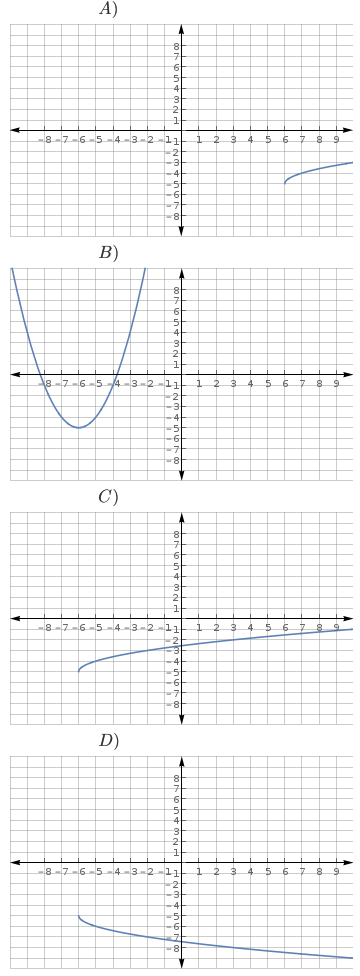26. (secA + 1)*(secA – 1) simplifies to tan²A. What trig identity is required to prove this?
This is a required question

27. A cubic contains the point (2,4), has a double root at -2, and a single root at 3. Identify the function.
This is a required question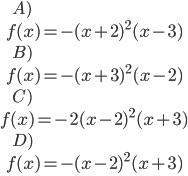28. Give the six trig functions in terms of opp, adj, and hyp.
This is a required question